# How to generate random character strings in a range in Excel?

Random data can be identified as random values, used to obtain some results. It can be used to perform simulations, to understand the pattern of different system components. It is useful when a user requires a large data set to evaluate some common feature. This article allows the user to generate a random string with three possible methods. The first method is based on the user-defined formula, the second method is based on the use of VBA code, and the final third method is based on the use of kutool.

## Example 1: To generate random character string in a range in excel by using the formula

### Step 1

This article guides user about the process of generating random string characters in excel by the user-defined formula. To understand the example thoroughly, consider the below given spreadsheet −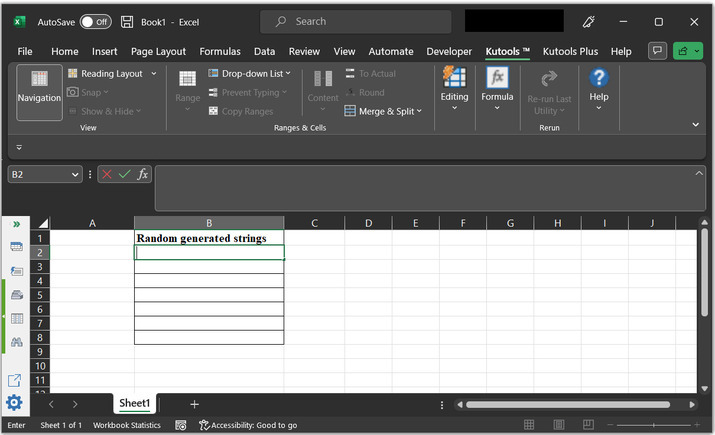### Step 2

In the worksheet, go to the B2 cell, and type the below given formula −

=CHAR(RANDBETWEEN(65,90))&CHAR(RANDBETWEEN(66,89))&RANDBETWEEN(10,99)


Snapshot for reference −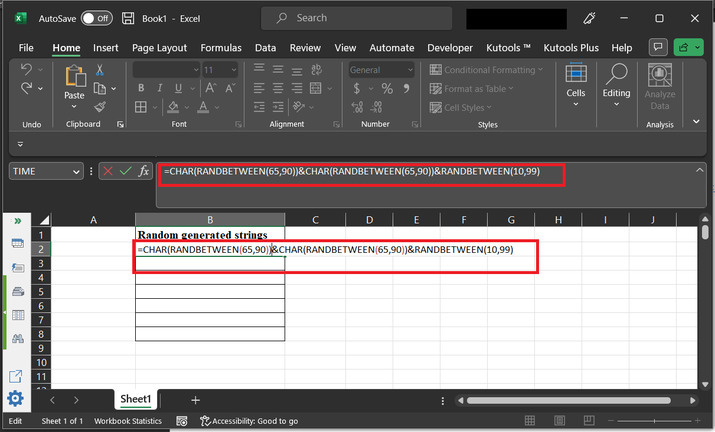### Explanation of formula

• CHAR is a function in Excel that converts a number into the corresponding character in the ASCII table. For example, CHAR (65) returns the character "A".

• RANDBETWEEN is another function in Excel that generates a random number between two specified values.

• The first CHAR (RANDBETWEEN (65,90)) generates a random uppercase letter between A and Z.

• The second CHAR (RANDBETWEEN (66,89)) generates another uppercase letter between B and Y.

• The third RANDBETWEEN (10,99) generates a random number between 10 and 99.

Putting these components together, the formula creates a string with two random uppercase letters and a random two-digit number.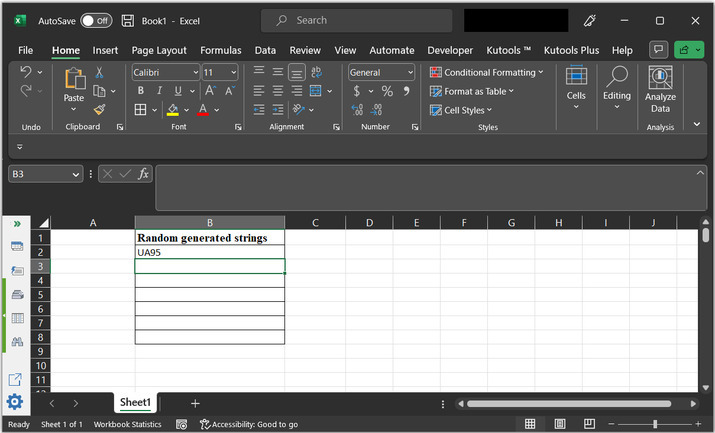### Step 3

This formula will generate results for the B2 cell. to copy the formula, to another cell, drag the “+” sign to the bottom of the cell.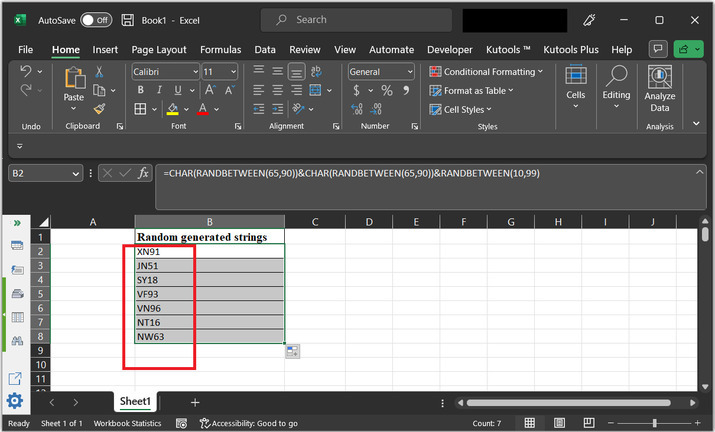## Example 2: To generate a random character string in a range in Excel by using the VBA code

### Step 1

This example uses the VBA code to generate random string data. To open the code area, go to the “Developer” tab, and choose “Visual Basic” under the “Code” section. Consider the below-given snapshot for reference −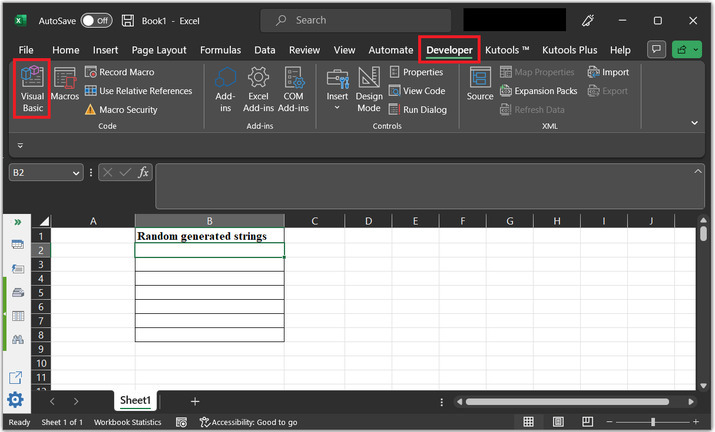### Step 2

This will open the “Microsoft Visual Basic for Applications” dialog box, as shown below −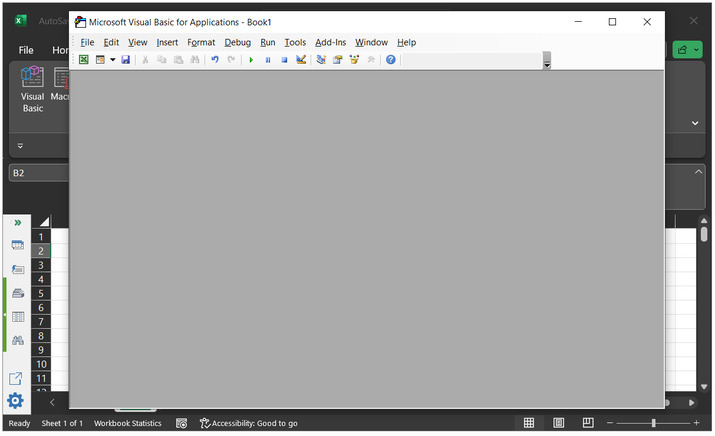### Step 3

In the dialog box, click on “Insert” tab and then choose “Module” option. As, depicted below −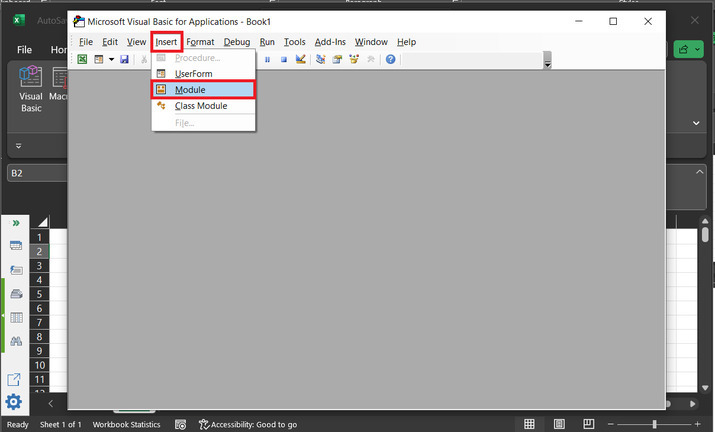### Step 4

This will open a code area. Type the below given code to the code area.

Code −

' define function header
Public Function Func_ranom(g1 As Integer, g2 As Integer)
' declare random variable
Dim ran As String
' volatile data
Application.Volatile
' calculating length
t_len = Int((g2 + 1 - g1) * Rnd + g1)
' do expression
Do
' calculating i variable
i = i + 1
' calculating randomize
Randomize
' calculating ran variable
ran = ran & Chr(Int((90) * Rnd + 36))
' loop variable
Loop Until i = t_len
' calling function
Func_ranom = ran
End Function


Please use proper code indentation to ensure proper execution of code. Snapshot of code area −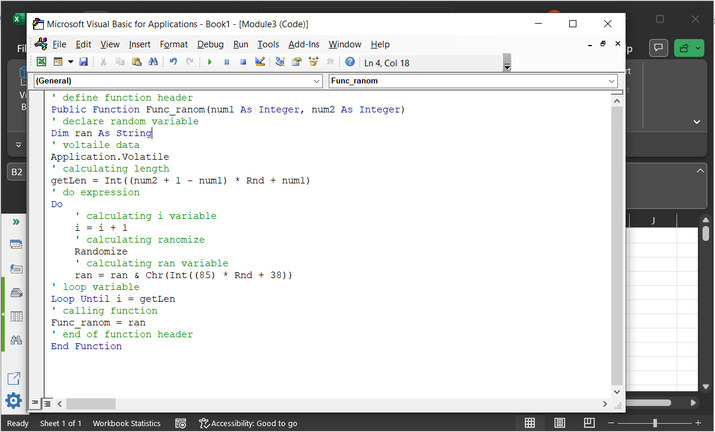### Step 5

Again, go to the excel sheet, and call the required method. Expression, to call method, “ =Func_ranom(5,10)”.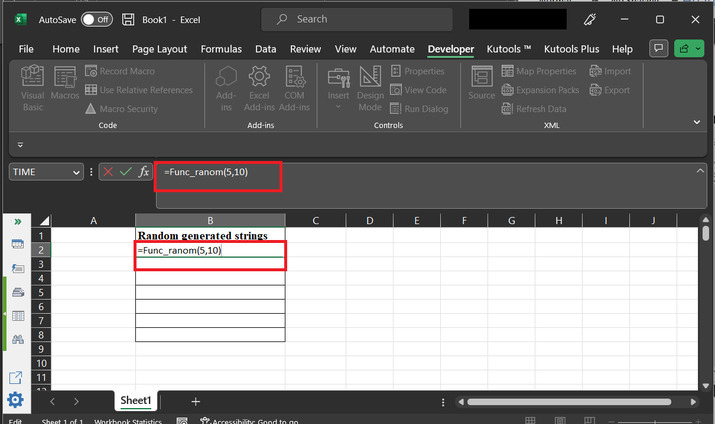### Step 6

Finally, generated results are depicted below. To copy the formula, to the rest rows, drag the “+” sign to the bottom.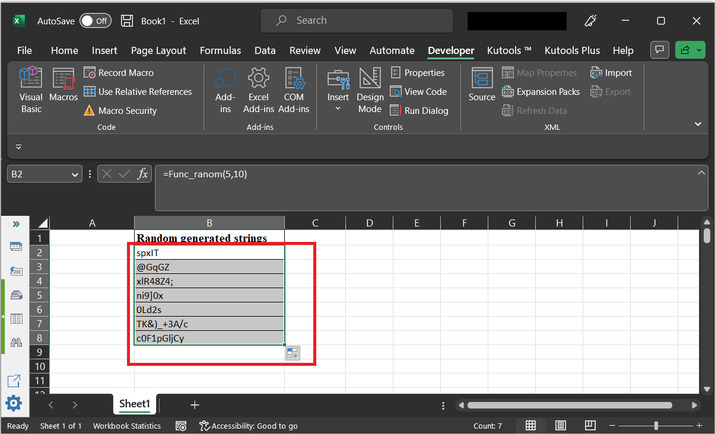## Example 3: To generate random character string in a range in excel by using the Kutool

### Step 1

Open the spreadsheet with the same beginning data. Go to the “Kutools” tab and select the “Editing” option. Under the “editing” section choose the option “Insert”. Further select the option “Insert Random Data”.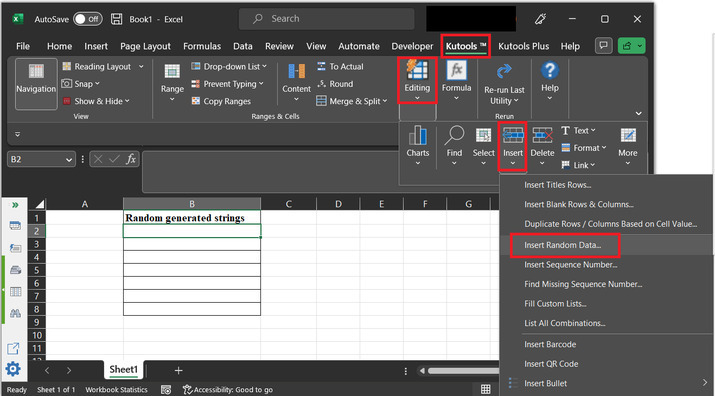### Step 2

This will open the “Insert Random Data” dialog box, as depicted below −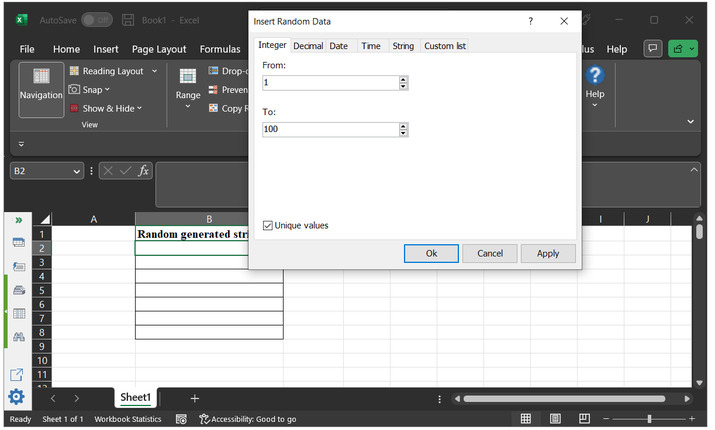### Step 3

In the opened dialog box, choose the tab “String”. Tick all the required options. Choose the required string length according to the requirement. For this case, will assume the string length as 4.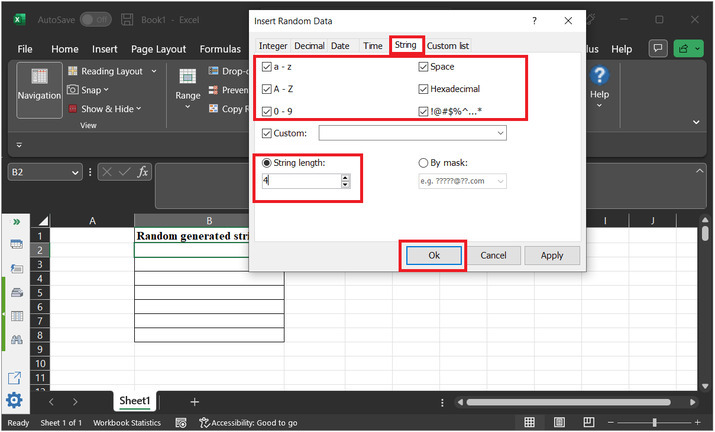### Step 4

The generated results are depicted below −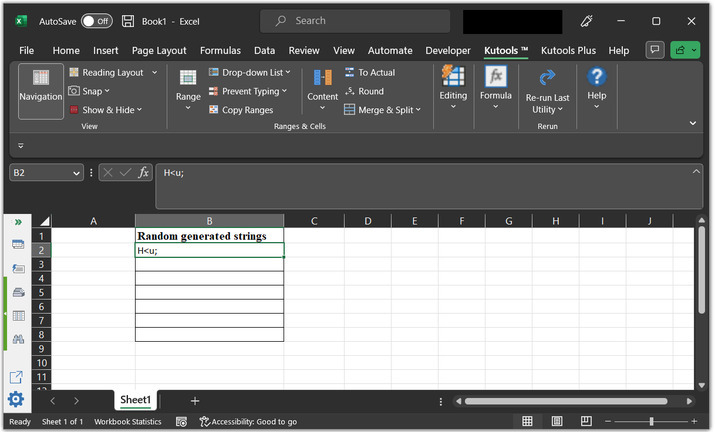## Conclusion

After the successful completion of this article, the user will be able to generate the string of random characters of any required length. This article briefs users with 3 different examples to achieve the same task. Users can use any task according to the need and ease in nature of using the data.

Updated on: 08-May-2023

1K+ Views